# Implementing k-Nearest Neighbors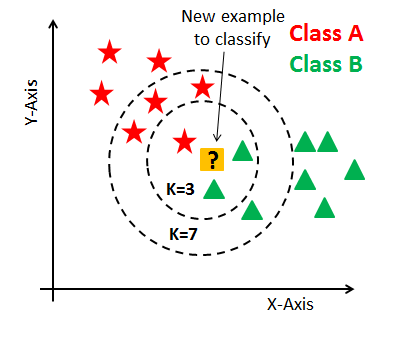Classification example. K=3 will choose triangle, while K=7 chooses the stars.
1. Calculate Euclidean Distance
2. Get Nearest Neighbors (Fit)
3. Make Predictions
4. Display!Euclidean Distance Example from my Github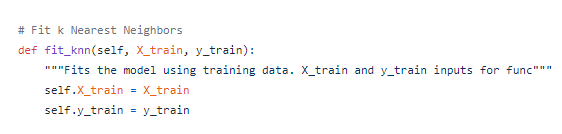Fitting the data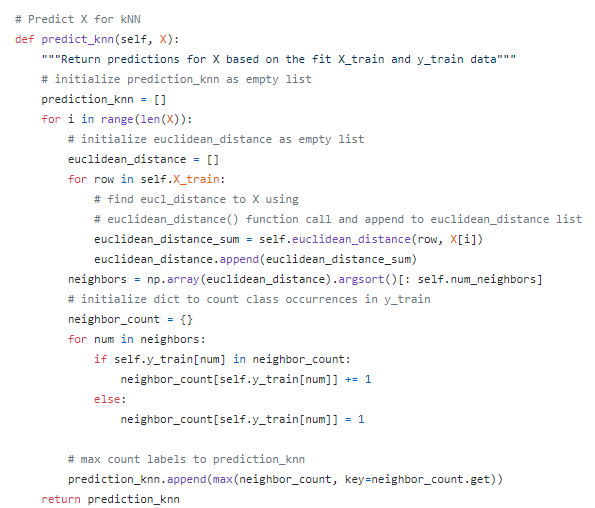The prediction section ties in the Euclidean Distance and Fit data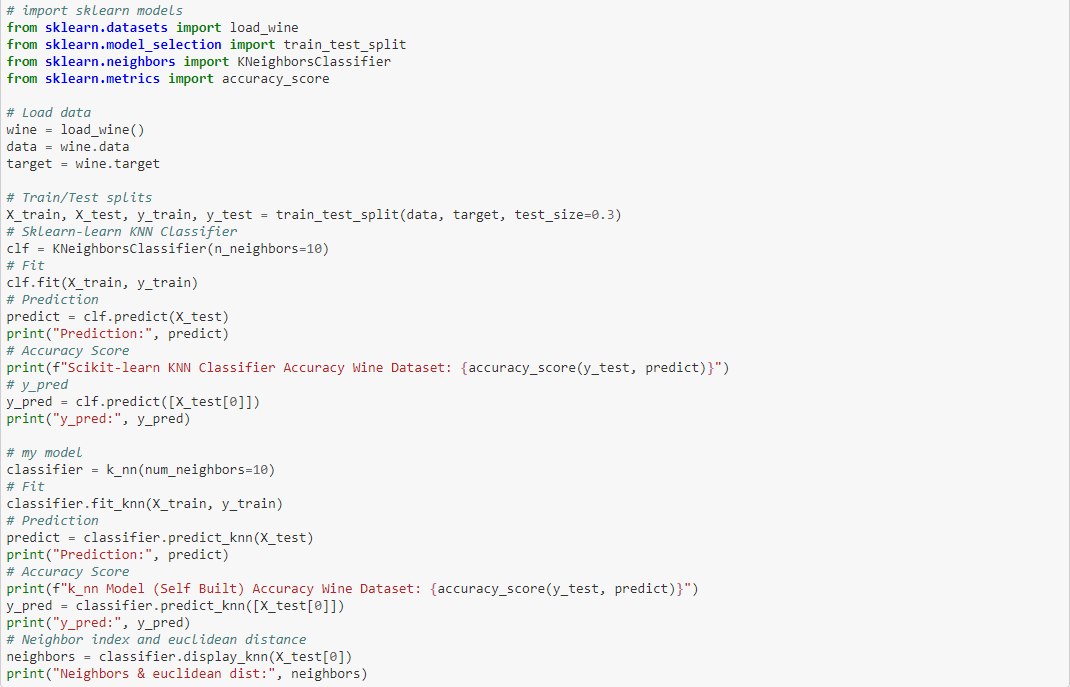Comparing sklearn model with the one written above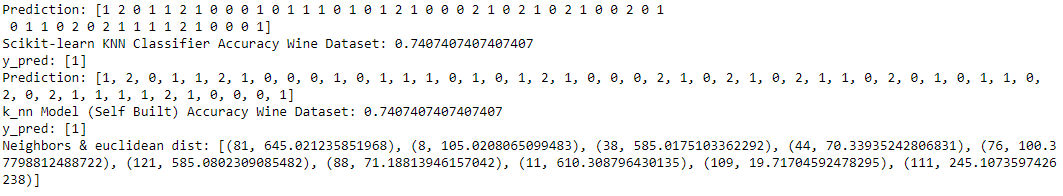Output of sklearn, then self built k-NN

--

--